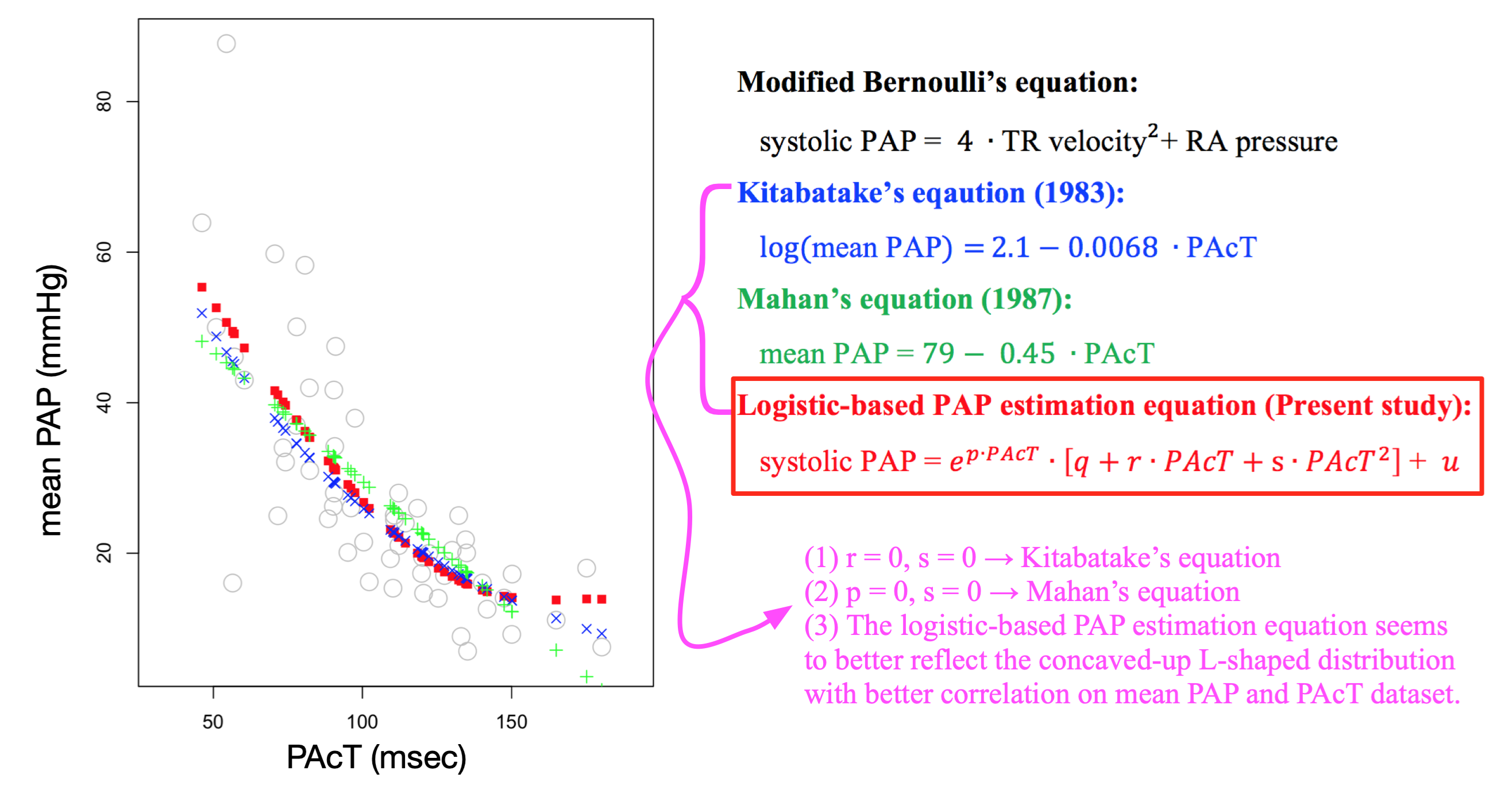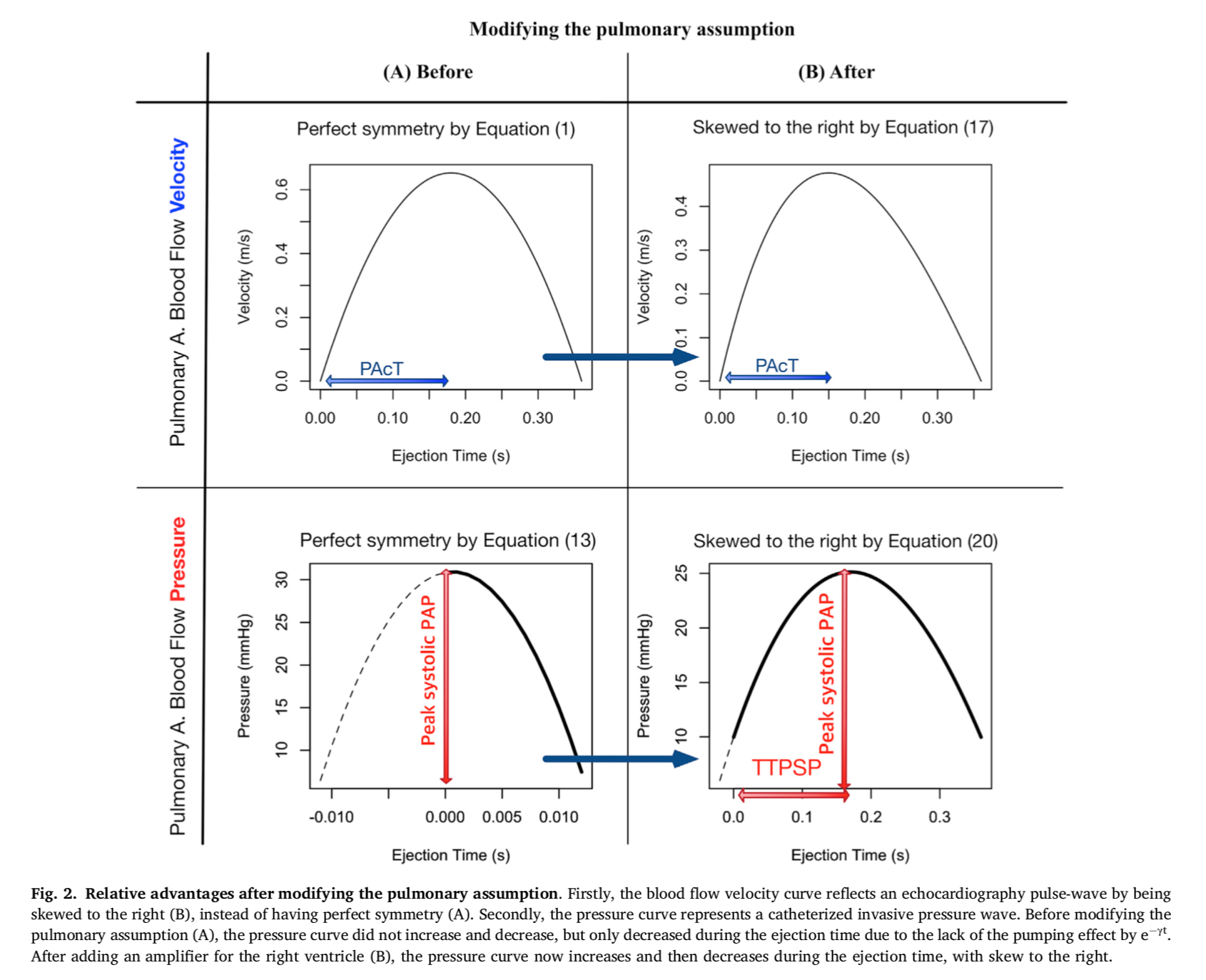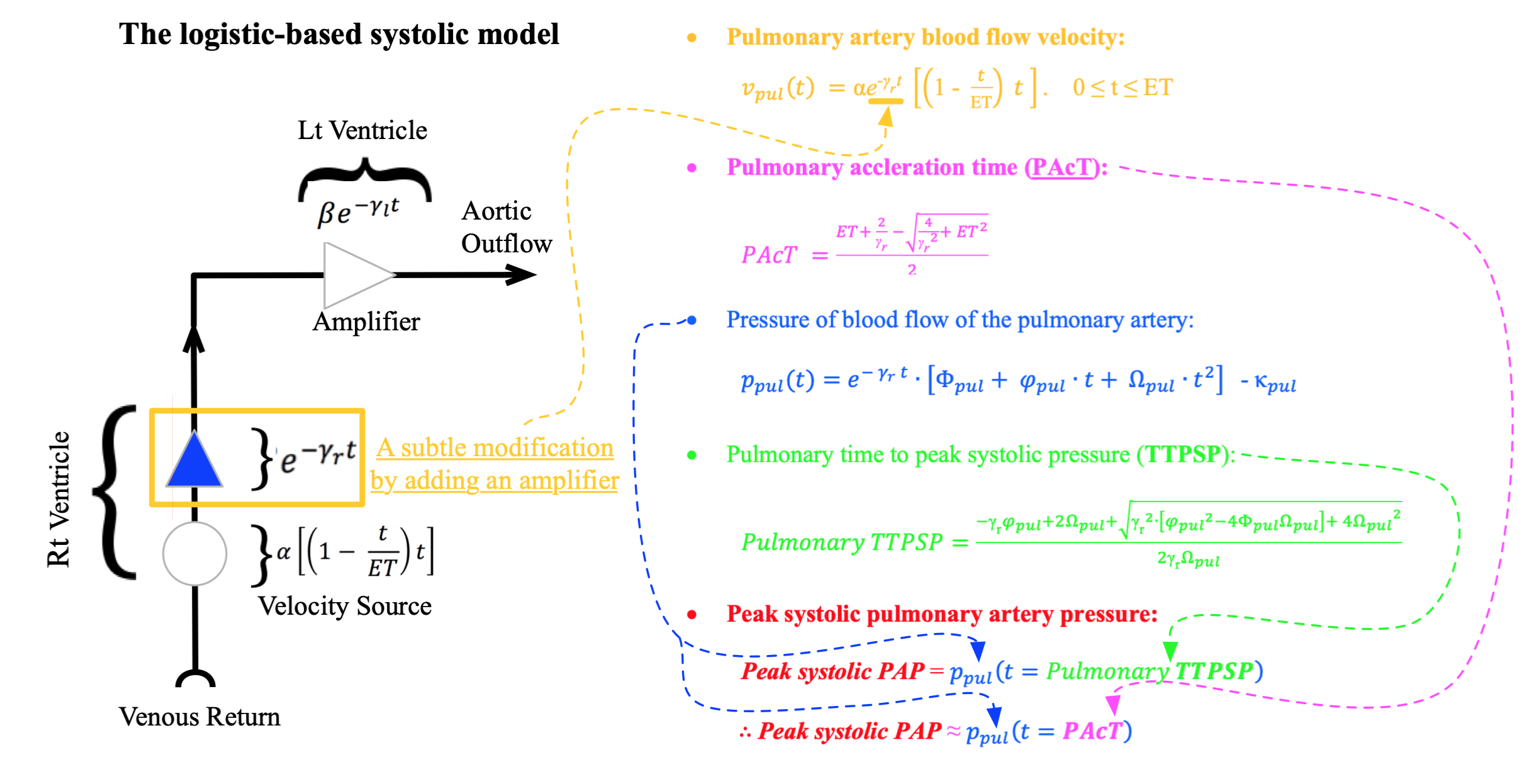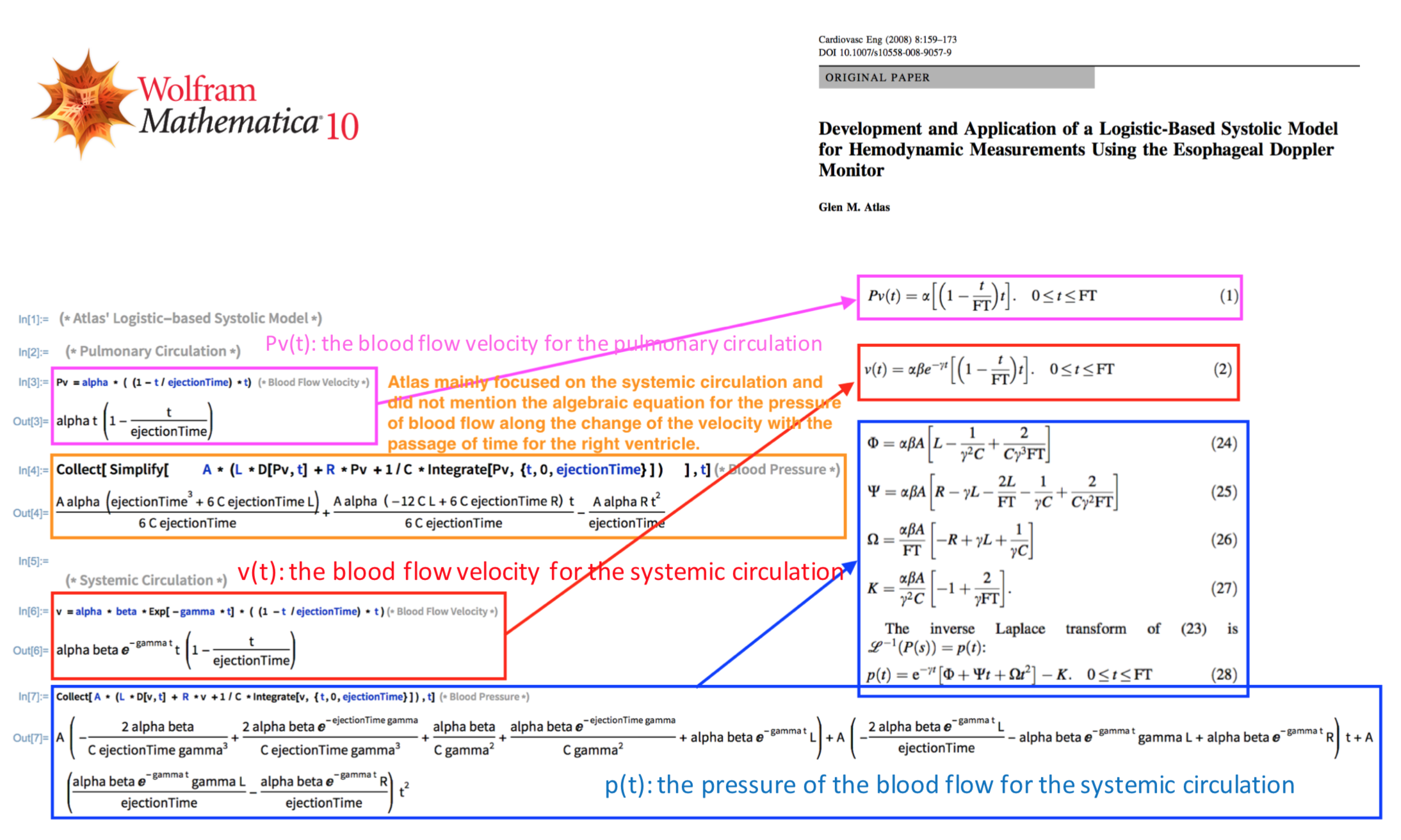Home Hemodynamic Simulation Resource: ECMO, Script Frank's Projects Parmar's methodology in dev: Download & Document, TMP Software Architecture in association with Robot

# Hemodynamic Simulation

A practical example of a clinical use of hemodynamic simulation, when inducing a logistic-based PAP estimation equation, based on a hemodynamic model (Frank, 2018):

 α   7.25 L   3.10 γr 1.90 ET 0.36 R   8.70 γl 4.25 Ap 5.90 C   3.30 γ   6.10 As 3.80 β   3.00
Canvas not supported

### Inducing Logistic-based PAP estimation equation(Frank, 2018):

• A logical ground for the relationship between systolic pulmonary artery pressure (PAP) and pulmonary acceleration time (PAcT) using transthoracic echocardiography was established based on a logistic-based systolic model.
• The logistic-based PAP estimation equation was deduced from the model to relate systolic PAP and PAcT.
• Results showed that the logistic-based PAP estimation equation was at least as accurate as previous equations with respect to previously published mean PAP versus PAcT values.### (0) Preface

Many great works of hemodynamics should better be reached and employed by clinicians more frequently and intuitively. Nonetheless, it may be difficult to work with the hemodynamic model itself due to multiple variables and complicated expressions, but as long as it can be visualized in order to better understand the meaning of each variable, it can be more readily applied to solve clinical questions. I will have intended to set examples of using the hemodynamic model and making a slight change if needed for a clinical application as a hemodyanmic software architect in association with device programming and machine learning, and certainly do not wish to position myself as a hemodynamic model creator.

### (1) Logistic-Based Systolic Model (Atlas, 2008) and Pulmonary Circulation

Logistic-based systolic model (Atlas, 2008) was developed to reflect aortic blood flow velocity and pressure that were observed by esophageal Doppler monitor (EDM). "As every model somehow simplifies the physiologies, any model may be modified or expanded for a certain purpose or application. This hemodynamic model requires a minor modification to better reflect the pulmonary circulation physiologies and to support the relationship between PAcT and PAP" (Frank, 2018).

#### LBSM has the following aspects that should be improved for the pulmonary circulation:

a) Blood flow velocity: Pv(t) = α∙((1 - t/ET)∙t)     Perfect symmetry -> Skewed to the right

Skewedness, observed by Echocardiography, is a key characteristic, which reflects the relationship between pulmonary acceleration time (PAcT) and pulmonary arterial pressure (PAP).

b) Blood flow pressure: v(t) = Φ + Ψ∙t + Ω∙t2     Perfect symmetry -> Skewed to the right

The overall skewed shape of pressure curve, observed by Catheterization, is critical in mathematically integrating area under the curve for diagnosing PAP per the definition of pulmonary hypertension.c) Correlation between PAP and PAcT: Pv'(t) = α∙(1-2/ET∙t)

According to LBSM (Atlas, 2008), since the blood flow velocity waveform is a quadratic function with a perfect symmetry with respect to t = ET/2, no correlation between PAP (pulmonary artery pressure) and PAcT (pulmonary arterial acceleration time) should be observed. This is, in fact, not the case, based on significantly high correlation between PAP and PAcT in (Kitabatake, 1983) and (Dabestani, 1987).

d) Assumption of LBSM

No pumping effect was previously assumed for the pulmonary circulation (Atlas, 2008). "Despite the fact that RV pumps weakly compared to LV, to better reflect pulmonary physiologies, such as the correlation between PAP and PAcT and the skeness of velocity and pressure curve of pulmonary circulation, it is necessary to assume a pumping effect on pulmonary circulation as well" (Frank, 2018).

A subtle modification was made by adding an amplifier for the pulmonary circulation. The right ventricle acts as a velocity source and an amplifier, whereas the left ventricle plays the role of an amplifier. In this model, er*t and el*t are employed to reflect the pumping effect of the right and left ventricles, respectively.e) Re-inducing the LBSM model, based on the subtly modified assumption, by Mathematica

Collect [ Simplify [A * (L * D[Pv, t] + R * Pv + 1/C * Integrate [Pv, {t, 0, ET}] ), t]### (2) Establishing Logistic-based PAP Estimation Equationa) Simulated results of the logistic-based systolic model

The model (Atlas, 2008) was modified to reflect pulmonary circulation physiology in relation to systemic circulation. Then, the model (Frank, 2018) could represent both circulation physiologies, by being capable of better resembling actual blood flow velocity and pressure curves.

b) Observational measurements of mammals, redrawn from Figure 4.2.2. of Li.

For pulmonary circulation, the difference between aortic acceleration time (AcT) and time to peak systolic pressure (TTPSP) is relatively small compared to that of the less compliant systemic circulation. Thus, the relationship between pulmonary acceleration time (PAcT) and PAP can be induced by substituting TTPSP, which requires invasive catheterized measurements, with PAcT, which can be measured by transthoracic echocardiography. Per the Equation: Systolic PAP = p(time), the systolic PAP can be approximately equal to the function of PAcT.

### (3) Evaluating Logistic-based PAP Estimation EquationThe mean PAP and PAcT pair values, gathered from Dabestani et al., Kitabatake et al., and Tousignant et al., were employed. The overall distribution between mean PAP and PAcT did not appear to be linear, but rather L-shaped. The predicted values for the linear Mahan's relationship, the logarithmic Kitabatake's relationship, and the proposed logistic-based relationship were represented, respectively, whose Pearson correlation coefficients were 0.749, 0.779, and 0.781 and the corresponding p-values were below 0.05.

## Acknowledgements

"We appreciate greatly that John K-J. Li and Glen M. Atlas read and edited the manuscript. We acknowledge that this article employs the existing logistic-based systolic model, developed by G.M.A, with a subtle modification to develop our logic and clinical application. Thus, out of our respect for the scrutinized work of G.M.A., the model in this article is still named as the logistic-based systolic model" (Frank, 2018).

© nGene Hemodynamic Research Center 2013
Project nGene.org® is a registered trademark.